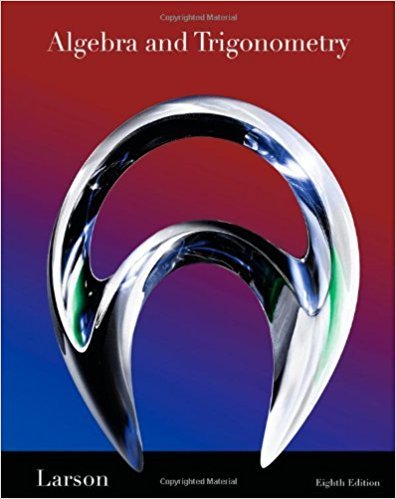×
×

# Solutions for Chapter 11.6: Counting Principles## Full solutions for Algebra and Trigonometry | 8th Edition

ISBN: 9781439048474Solutions for Chapter 11.6: Counting Principles

Solutions for Chapter 11.6
4 5 0 357 Reviews
18
0
##### ISBN: 9781439048474

Since 97 problems in chapter 11.6: Counting Principles have been answered, more than 47289 students have viewed full step-by-step solutions from this chapter. This textbook survival guide was created for the textbook: Algebra and Trigonometry, edition: 8. Chapter 11.6: Counting Principles includes 97 full step-by-step solutions. Algebra and Trigonometry was written by and is associated to the ISBN: 9781439048474. This expansive textbook survival guide covers the following chapters and their solutions.

Key Math Terms and definitions covered in this textbook
• Cayley-Hamilton Theorem.

peA) = det(A - AI) has peA) = zero matrix.

• Eigenvalue A and eigenvector x.

Ax = AX with x#-O so det(A - AI) = o.

• Fast Fourier Transform (FFT).

A factorization of the Fourier matrix Fn into e = log2 n matrices Si times a permutation. Each Si needs only nl2 multiplications, so Fnx and Fn-1c can be computed with ne/2 multiplications. Revolutionary.

• Free columns of A.

Columns without pivots; these are combinations of earlier columns.

• Hessenberg matrix H.

Triangular matrix with one extra nonzero adjacent diagonal.

• Kronecker product (tensor product) A ® B.

Blocks aij B, eigenvalues Ap(A)Aq(B).

• Multiplicities AM and G M.

The algebraic multiplicity A M of A is the number of times A appears as a root of det(A - AI) = O. The geometric multiplicity GM is the number of independent eigenvectors for A (= dimension of the eigenspace).

• Particular solution x p.

Any solution to Ax = b; often x p has free variables = o.

• Pivot columns of A.

Columns that contain pivots after row reduction. These are not combinations of earlier columns. The pivot columns are a basis for the column space.

• Polar decomposition A = Q H.

Orthogonal Q times positive (semi)definite H.

• Positive definite matrix A.

Symmetric matrix with positive eigenvalues and positive pivots. Definition: x T Ax > 0 unless x = O. Then A = LDLT with diag(D» O.

• Pseudoinverse A+ (Moore-Penrose inverse).

The n by m matrix that "inverts" A from column space back to row space, with N(A+) = N(AT). A+ A and AA+ are the projection matrices onto the row space and column space. Rank(A +) = rank(A).

• Row picture of Ax = b.

Each equation gives a plane in Rn; the planes intersect at x.

• Special solutions to As = O.

One free variable is Si = 1, other free variables = o.

• Stiffness matrix

If x gives the movements of the nodes, K x gives the internal forces. K = ATe A where C has spring constants from Hooke's Law and Ax = stretching.

• Subspace S of V.

Any vector space inside V, including V and Z = {zero vector only}.

• Triangle inequality II u + v II < II u II + II v II.

For matrix norms II A + B II < II A II + II B II·

• Vandermonde matrix V.

V c = b gives coefficients of p(x) = Co + ... + Cn_IXn- 1 with P(Xi) = bi. Vij = (Xi)j-I and det V = product of (Xk - Xi) for k > i.

• Vector v in Rn.

Sequence of n real numbers v = (VI, ... , Vn) = point in Rn.

• Wavelets Wjk(t).

Stretch and shift the time axis to create Wjk(t) = woo(2j t - k).

×